# The SEQDESIGN Procedure

### Boundary Scales

The boundaries computed by the SEQDESIGN procedure are applied to test statistics computed during the analysis, and so generally, the scale you select for the boundaries is determined by the scale of the statistics that you will be using.

The following scales are available in the SEQDESIGN procedure:

• MLE, maximum likelihood estimate

• standardized Z

• score statistic S

• p-value

These scales are all equivalent for a given set of boundary values—that is, there exists a unique transformation between any two of these scales. If you know the boundary values in terms of statistics from one scale, you can uniquely derive the boundary values of statistics for other scales. You can specify the scale with the BOUNDARYSCALE= option; the default is BOUNDARYSCALE=STDZ, the standardized Z scale.

You can also select the boundary scale to better examine the features of an individual group sequential design or to compare features among multiple designs. For example, with the standardized Z scale, the boundary values for the Pocock design are identical across all stages, and the O’Brien-Fleming design has boundary values (in absolute value) that decrease over the stages.

The remaining section demonstrates the transformations from one scale to the other scales. If the maximum likelihood estimate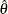is computed by the analysis, then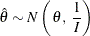where I is the Fisher information if it does not depend on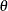. Otherwise, I is either the expected Fisher information evaluated ator the observed Fisher information. See the section Maximum Likelihood Estimator for a detailed description of these statistics.

With the MLE statistic, the corresponding standardized Z statistic is computed as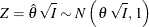and the corresponding score statistic is computed as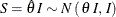Similarly, if a score statistic S is computed by the analysis, then with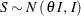where I is the information, either an expected Fisher information (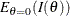or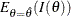) or an observed Fisher information (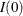or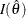).

The corresponding standardized Z statistic is computed as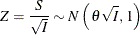and the corresponding MLE-scaled statistic is computed as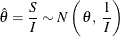With a standardized normal Z statistic, the corresponding fixed-sample nominal p-value depends on the type of alternative hypothesis. With an upper alternative, the nominal p-value is defined as the one-sided p-value under the null hypothesis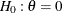with an upper alternative: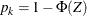With a lower alternative or a two-sided alternative, the nominal p-value is defined as the one-sided p-value under the null hypothesiswith a lower alternative: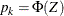which is an increasing function of the standardized Z statistic (Emerson, Kittelson, and Gillen 2005, p. 12).

The BOUNDARYSCALE= MLE, STDZ, SCORE, and PVALUE options display the boundary values in the MLE, standardize Z, score, and p-value scales, respectively. For example, suppose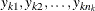are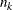observations of a response variable Y in a data set from a normal distribution with an unknown meanand a known variance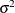. Then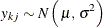for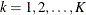, where K is the number of groups andis the number of observations at group k.

If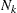is the cumulative number of observations for the first k groups, then the sample mean from theseobservations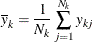has a normal distribution with meanand variance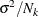: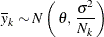To test the null hypothesis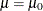,, where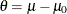can be used. The MLE ofis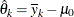and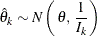where the information is the inverse of the variance of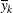,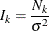The corresponding standardized Z statistic is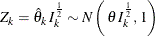The score statistic in the SEQDESIGN procedure is then given by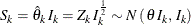For a null hypothesis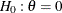with an upper alternative, the nominal p-value of the standardized Z statistic is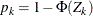. For a null hypothesiswith a lower alternative or a two-sided alternative, the nominal p-value of the standardized Z statistic is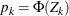.